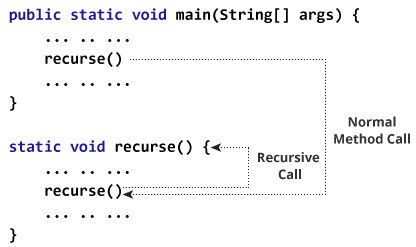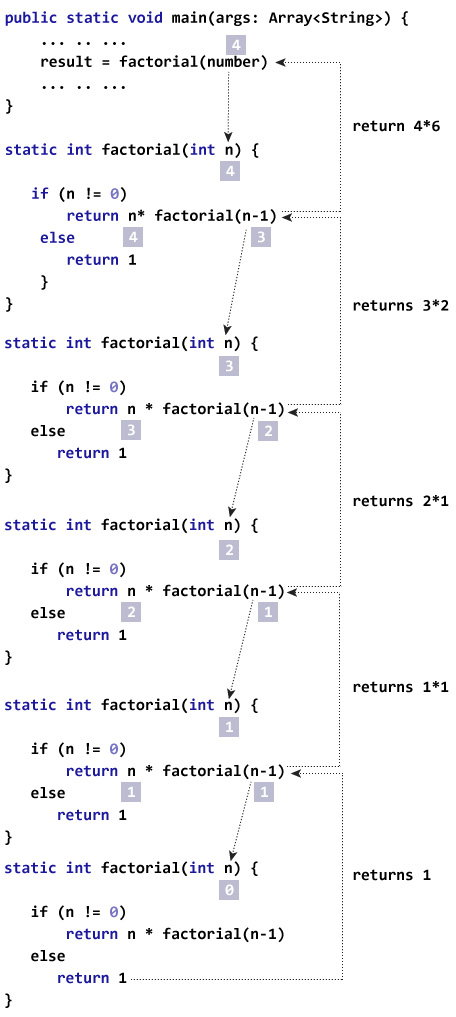Java Recursion

# Java Recursion

A method that calls itself is known as a recursive method. And, this technique is known as recursion.

A physical world example would be to place two parallel mirrors facing each other. Any object in between them would be reflected recursively.

### How recursion works?In the above program, `recurse()` method is called from inside the main method at first (normal method call).

Also, `recurse()` method is called from inside the same method, `recurse()`. This is a recursive call.

The recursion continues until some condition is met to prevent it from execution. If not, infinite recursion occurs.

Hence, to prevent infinite recursion, if...else statement (or similar approach) can be used where one branch makes the recursive call and other doesn't.

## Example: Factorial of a Number Using Recursion

``````class Factorial {

static int factorial( int n ) {
if (n != 0)
return n * factorial(n-1); // recursive call
else
return 1;
}

public static void main(String[] args) {
int number = 4, result;
result = factorial(number);
System.out.println(number + " factorial = " + result);
}
}``````

When you run above program, the output will be:

`4 factorial = 24`

Initially, `factorial()` is called from the `main()` method with number passed as an argument.

Inside `factorial()` method, the value of n is 4 initially. During the next recursive call, 3 is passed to the `factorial()` method. This process continues until n is equal to 0.

When n is equal to 0, `if` condition fails and the `else` part is executed which returns 1, and accumulated result is passed to the `main()` method.

This figure will give you a better idea on how the above program works.